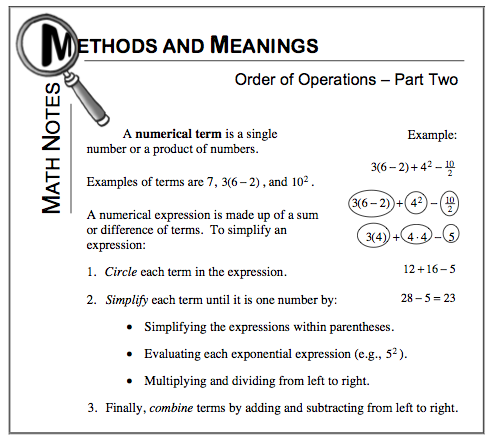### Home > MC1 > Chapter 7 > Lesson 7.2.4 > Problem7-85

7-85.

Simplify each of the following expressions.

1. $\frac { 7 \cdot 3 + 5 } { 2 }$

1. 3 + 8 ÷ 4 − 1

1. 2(12 − 4) + 4

2. 4(9 + 3) + 10 ÷ 2

For parts (a) through (d), refer to the Math Notes box below. Be sure to follow the Order of Operations!Try circling the terms first to make simplifying the expressions easier!

After circling the terms, always remember to work from left to right.
And be sure to simplify expressions within parentheses first!

The answer to part (c) is 20 and the answer to part (d) is 53. Remember to show all of your work!